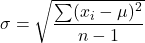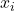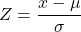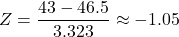A sample of 10 packs of a brand of chewing gum was taken. Each pack was weighed and their weights, in grams, are shown.

Question

A sample of 10 packs of a brand of chewing gum was
taken. Each pack was weighed and their weights, in
grams, are shown.
What is the Z-score for the pack of gum weighing 43
grams?
01.13
43.0, 43.7, 49.6, 46.9, 47.6, 45.4, 51.2, 48.0, 40.5, 49.1
11.05
01.05
01.13

in progress 0
5 months 2021-08-16T04:36:05+00:00 1 Answers 2 views 0

The z-score is approximately -1.05

Step-by-step explanation:

The given data of the weights of the packs of chewing gum are;

43.0, 43.7, 49.6, 46.9, 47.6, 45.4, 51.2, 48.0, 40.5, and 49.1

The number of chewing gums in the sample, n = 10

The sum of the weights of the chewing gums is therefore;

43.0+43.7+49.6+46.9+47.6+45.4+51.2+48.0+40.5+49.1 = 465

The average weight is given as follows;

The average weight of the chewing gums = (The sum of the weights of the chewing gums)/(The number chewing gums)

The average weight, μ, of the chewing gums = (465)/(10) = 46.5

The standard deviation, σ, is given by the formula;Where;= Each individual chewing gum weight values

With the standard deviation formula in Excel, we have;

σ ≈ 3.323 grams

The z-score, z, is given by the following formula;Therefore, the z-score of 43 is given as follows;